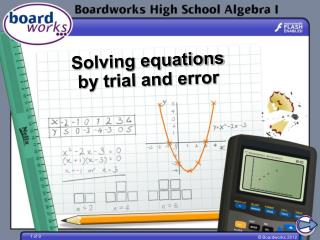DownloadDownload PresentationSolving equations by trial and error

Solving equations by trial and error

Télécharger la présentationSolving equations by trial and error

- - - - - - - - - - - - - - - - - - - - - - - - - - - E N D - - - - - - - - - - - - - - - - - - - - - - - - - - -
Presentation Transcript

1. Solving equations by trial and error

2. Trial and error Sometimes we know an approximate value for a solution of an equation, but we need to find it to a greater degree of accuracy. This can happen when we have seen a graph and can read off an approximate value for a root of a function or the intersection of two functions. We can improve the accuracy of our answer by substituting successive approximations into the equation and seeing whether they make it true. This method of finding a solution is called trial and error.

3. Tennis Dana hits a ball so that its height can be modeled by the equation h = –16t2 + 8t + 4, where h is height in feet and t is time in seconds since Dana hit it. The ball hits the ground after less than a second. Find this time to the nearest hundredth. When the ball hits the ground, its height is 0 feet. We need to solve the equation 0 = –16t2 + 8t + 4. As x is the time since Dana hit the ball, we are only interested in positive values for t. We need a value of t such that 0 ≤ t < 1.

4. Tennis solution x –16x2 + 8x + 4 ≈0? 1 -4 too low 0.9 -1.76 too low 0.8 0.16 too high 0.81 -.0176 too low 0.805 0.0716 too high This shows the solution is between 0.805 and 0.810, so it is 0.81 to the nearest hundredth. The ball hits the ground 0.81 seconds after Dana hit it.

5. Intersection Trial and error can also be used to find the intersection of two functions. The functions y = 3x3 + x2 + x + 7 and y = –2x3 + x2 – 68 intersect between x = 2 and x = 3. Find the x-coordinate of the point of intersection, correct to 2 decimal places. Write the functions as equal to each other: 3x3 + x2 + x – 7 = –2x3 + x2 + 68 Rearrange so all variables are on the same side: 3x3 + 2x3 + x2 – x2 + x = 68 + 7 5x3 + x = 75 Now solve this equation by trial and error.

6. Intersection solution x 5x3 + x ≈75? 2.5 80.63 too high 2.4 71.52 too low 2.45 75.98 too high 2.44 75.07 too high 2.43 74.17 too low 2.435 74.62 too low The functions y = 3x3 + x2 + x + 7 and y = –2x3 + x2 – 68 intersect at x = 2.44, correct to 2 decimal places.